# Excel：LOOKUP函数的经典用法

2018/02/28 14:47

1、逆向查询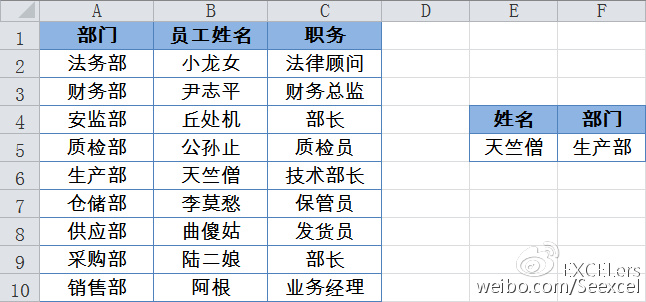​现在要根据E5单元格中的员工姓名，在这个信息表中查询属于哪个部门，也就是咱们常说的逆向查询，就可以使用LOOKUP函数了。

F5单元格输入以下公式：

=LOOKUP(1,0/(B2:B10=E5),A2:A10)

=LOOKUP(1,0/(条件),目标区域或数组)

=LOOKUP(1,0/((条件1)*( 条件2)* ( 条件N)),目标区域或数组)

2、查询A列中的最后一个文本

=LOOKUP("々",A:A )

"々"通常被看做是一个编码较大的字符，它的输入方法为<Alt 41385>组合键。

=LOOKUP("座",A:A )

​3、查询A列中的最后一个数值

=LOOKUP(9E307,A:A)

9E307被认为是接近Excel规范与限制允许键入最大数值的数，用它做查询值，可以返回一列或一行中的最后一个数值。

=LOOKUP(1,0/(A:A<>""),A:A)

​4、根据简称查询全称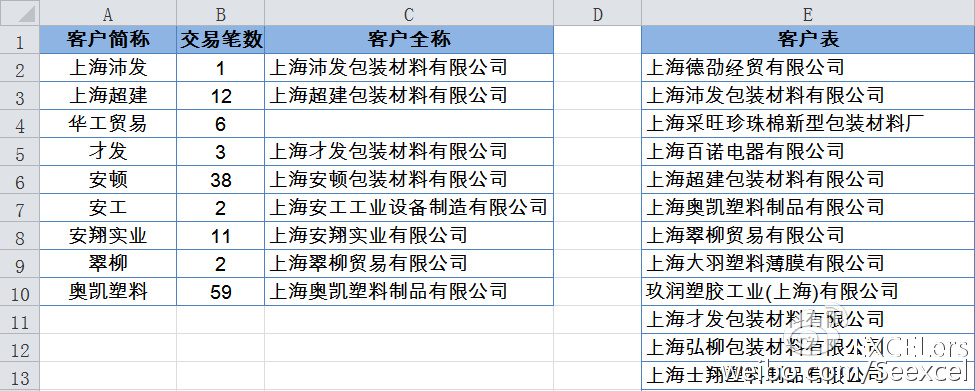​C2单元格输入以下公式，可得到“上海沛发”的客户全称“上海沛发包装材料有限公司”。

=IFERROR(LOOKUP(1,0/FIND(A2,E$2:E$13),E$2:E$13),"")

​5、多个区间的条件判断

50分以下的为“很差”

50-59分的为“差”

60-74分的为“一般”

75-85分的为“较好”

86-95分的为“优秀”

96分及以上的为“能手”。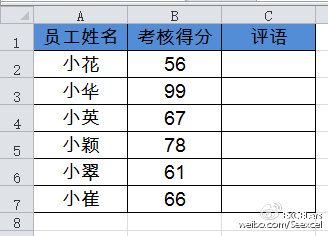​这种多个区间的判断，如果需要判断的条件和区间都很多，再使用IF函数来计算，估计会把自己都转晕了。

C2单元格输入以下公式，向下复制即可。

=LOOKUP(B2,{0,50,60,75,86,96;"很差","差","一般","较好","优秀","能手"})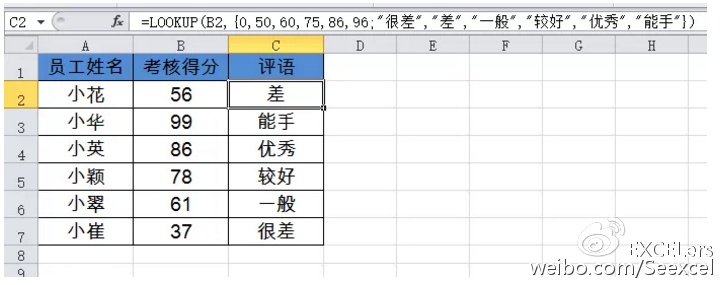​除此之外，LOOKUP函数还被用于带有合并单元格的汇总计算，以及单元格中数值字段的提取等等，这些内容咱们留到以后慢慢再说。

=LOOKUP(1,0/(条件区域=条件),查询区域)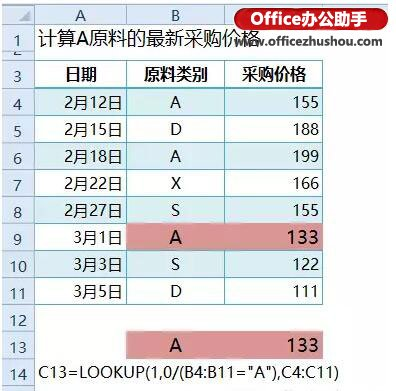=LOOKUP(1,0/((条件区域1=条件1)*(条件区域2=条件2)),查询区域)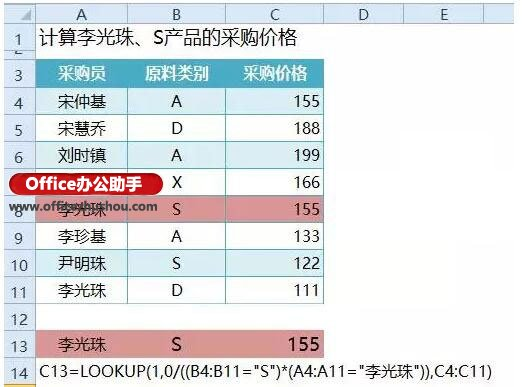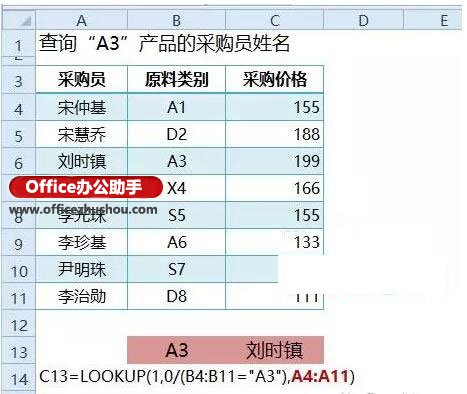=LOOKUP(1,0/(条件区域<>""),查询区域)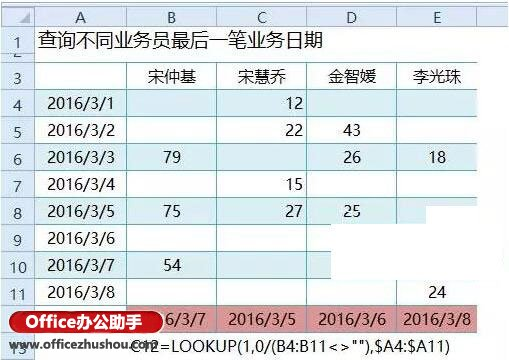=LOOKUP(1,0/B4:B11,$A4:$A11)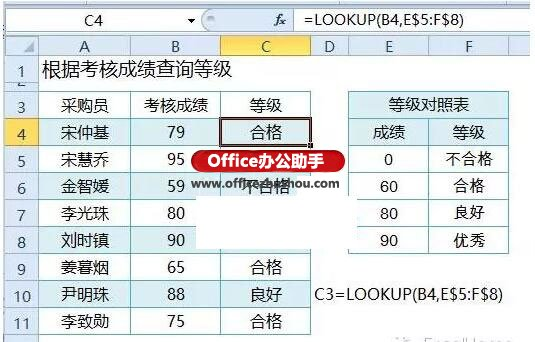A2公式为

=-LOOKUP(1,-LEFT(A2,ROW($1:$99)))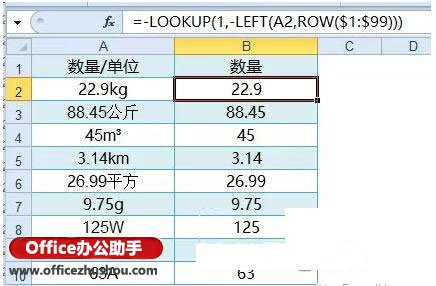LOOKUP函数使用1作为查询值，在由负数、0和错误值构成的数组中，忽略错误值提取最后一个等于或小于1的数值。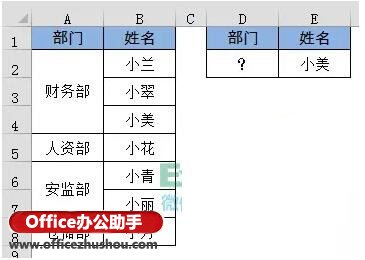0
0 收藏

### 作者的其它热门文章0 评论
0 收藏
0